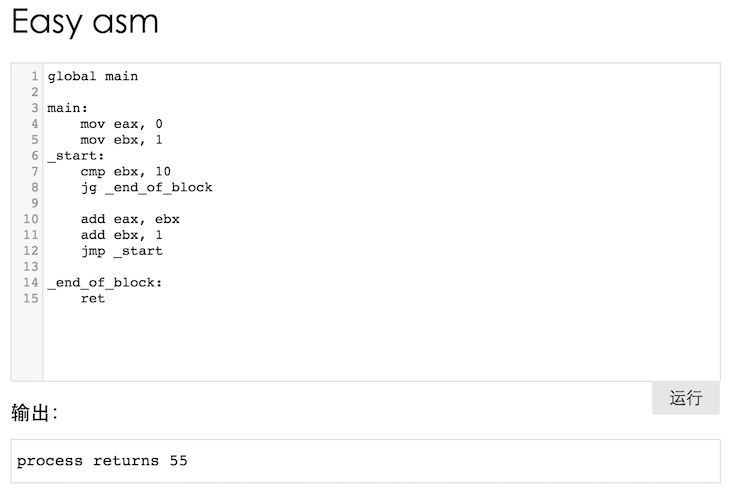• 比较
• 跳转

拆散循环结构

• 程序在往回跳转

(Talk is chip, show your code!)

``````int sum = 0;
int i = 1;
while( i <= 10 ) {
sum = sum + i;
i = i + 1;
}``````

• 1. 比较i和10的大小
• 2. 如果i <= 10则执行代码块，并回到(1)
• 3. 如果不满足 i <= 10，则跳过代码块

``````int sum = 10;
int i = 1;

_start:
if( i <= 10 ) {
sum = sum + i;
i = i + 1;
goto _start;
}``````

``````if ( a > 10 ) {
// some code
}``````

``````cmp eax, 10
jle out_of_block

; some code

out_of_block:``````

``````if( a <= 10 ) goto out_of_block;

// some code

out_of_block:``````

• 山寨版中，if块里只需要放一条跳转语句即可
• 山寨版中，if里的条件是反过来的
• 山寨版中，跳转语句的功能是跳过“正宗C代码”的if块

``````int sum = 10;
int i = 1;

_start:
if( i > 10 ) {
goto _end_of_block;
}

sum = sum + i;
i = i + 1;
goto _start;

_end_of_block:``````

``````int sum = 0;
int i = 1;
while( i <= 10 ) {
sum = sum + i;
i = i + 1;
}``````

• 所有if块中都仅有一条goto语句，别的啥都没了

用汇编写出循环

``````int sum = 10;
int i = 1;

_start:
if( i > 10 ) {
goto _end_of_block;
}

sum = sum + i;
i = i + 1;
goto _start;

_end_of_block:``````

``````global main

main:
mov eax, 0
mov ebx, 1
_start:
cmp ebx, 10
jg _end_of_block

jmp _start

_end_of_block:
ret``````

• 单条goto语句可以直接用jmp语句替代
• if和goto组合的语句块可以用cmp和j*指令的组合替代

• 将while循环拆解成只有if和goto的形式
• 将if形式的语句拆解成if块中仅有一行goto语句的形式
• 从前往后逐行翻译成汇编语言

其它循环呢？

``````int i = 1;
int sum = 0;
for(i = 0; i <= 10; i ++) {
sum = sum + i;
}
int sum = 0;
int i = 1;
while( i <= 10 ) {
sum = sum + i;
i = i + 1;
}``````

• 比较
• 跳转